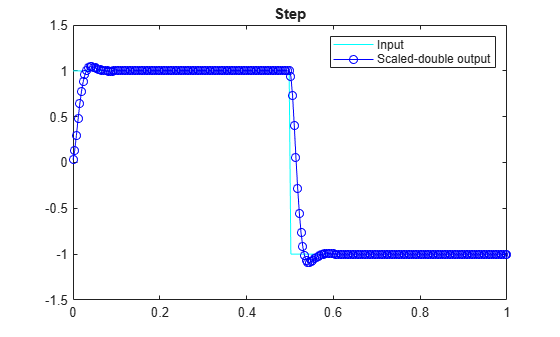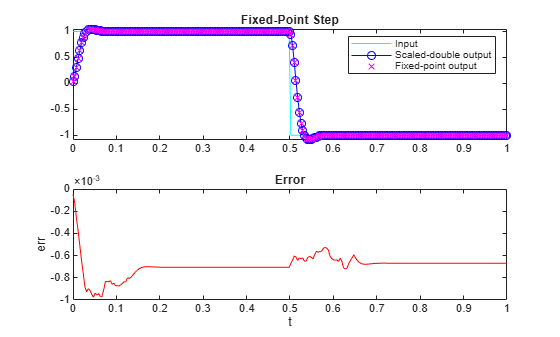# Set Data Types Using Min/Max Instrumentation

In this example, you set fixed-point data types by instrumenting MATLAB® code for min/max logging then use the tools to propose data types. You use the `buildInstrumentedMex` function to build the MEX function with instrumentation enabled, then use the `showInstrumentationResults` to show instrumentation results and the `clearInstrumentationResults` funciton to clear instrumentation results.

### Define the Unit Under Test

The function that you convert to fixed-point in this example is a second-order direct-form 2 transposed filter. You can substitute your own function in place of this one to reproduce these steps in your own work.

```function [y,z] = fi_2nd_order_df2t_filter(b,a,x,y,z) for i=1:length(x) y(i) = b(1)*x(i) + z(1); z(1) = b(2)*x(i) + z(2) - a(2) * y(i); z(2) = b(3)*x(i) - a(3) * y(i); end end ```

For a MATLAB® function to be instrumented, it must be suitable for code generation. For information on code generation, see the `buildInstrumentedMex` reference page. A MATLAB® Coder™ license is not required to use the `buildInstrumentedMex` function.

In the function `fi_2nd_order_df2t_filter`, the variables `y` and `z` are used as both inputs and outputs. This is an important pattern because:

• You can set the data type of `y` and `z` outside the function, thus allowing you to re-use the function for both fixed-point and floating-point types.

• The generated C code will create `y` and `z` as references in the function argument list.

### Use Design Requirements to Determine Data Types

In this example, the requirements of the design determine the data type of input `x`. These requirements are signed, 16-bit, and fractional.

```clearvars N = 256; x = fi(zeros(N,1),1,16,15);```

The requirements of the design also determine the fixed-point math for a DSP target with a 40-bit accumulator. This example uses floor rounding and wrap on overflow to produce efficient generated code.

```F = fimath('RoundingMethod','Floor',... 'OverflowAction','Wrap',... 'ProductMode','KeepLSB',... 'ProductWordLength',40,... 'SumMode','KeepLSB',... 'SumWordLength',40);```

The following coefficients correspond to a second-order lowpass filter created by

```[num,den] = butter(2,0.125) ```

The values of the coefficients influence the range of the values that will be assigned to the filter output and states.

```num = [0.0299545822080925 0.0599091644161849 0.0299545822080925]; den = [1 -1.4542435862515900 0.5740619150839550];```

The data type of the coefficients, determined by the requirements of the design, are specified as 16-bit word length and scaled to best-precision. To create `fi` objects from constant coefficients:

1. Cast the coefficients to `fi` objects using the default round-to-nearest and saturate on overflow settings, which gives the coefficients better accuracy.

```b = fi(num,1,16); a = fi(den,1,16);```

2. Attach `fimath` with floor rounding and wrap on overflow settings to control arithmetic, which leads to more efficient C code.

```b = setfimath(b,F); a = setfimath(a,F);```

### Use Values of the Coefficients and Inputs to Determine Data Types

The values of the coefficients and values of the inputs determine the data types of output `y` and state vector `z`. Create them with a scaled double datatype so their values will attain full range and you can identify potential overflows and propose data types.

```yisd = fi(zeros(N,1),1,16,15,'DataType','ScaledDouble','fimath',F); zisd = fi(zeros(2,1),1,16,15,'DataType','ScaledDouble','fimath',F);```

### Instrument the MATLAB Function as a Scaled-Double MEX Function

To instrument the MATLAB® code, you create a MEX function from the MATLAB® function using the `buildInstrumentedMex` function. The inputs to `buildInstrumentedMex` are the same as the inputs to `fiaccel`, but `buildInstrumentedMex` has no `fi`-object restrictions. The output of `buildInstrumentedMex` is a MEX function with instrumentation inserted. When the MEX function is run, the simulated minimum and maximum values are recorded for all named variables and intermediate values.

Use the `'-o'` option to name the MEX function that is generated. If you do not use the `'-o'` option, then the MEX function is the name of the MATLAB® function with `'_mex'` appended. You can also name the MEX function the same as the MATLAB® function, but you need to remember that MEX functions take precedence over MATLAB® functions and so changes to the MATLAB® function will not run until either the MEX function is re-generated, or the MEX function is deleted and cleared.

Hard-code the filter coefficients into the implementation of this filter by passing them as constants to the `buildInstrumentedMex` function.

```buildInstrumentedMex fi_2nd_order_df2t_filter ... -o filter_scaled_double ... -args {coder.Constant(b),coder.Constant(a),x,yisd,zisd}```

### Test Bench with Chirp Input

The test bench for this system is set up to run chirp and step signals. In general, test benches for systems should cover a wide range of input signals.

The first test bench uses a chirp input. A chirp signal is a good representative input because it covers a wide range of frequencies.

```t = linspace(0,1,N); % Time vector from 0 to 1 second f1 = N/2; % Target frequency of chirp set to Nyquist xchirp = sin(pi*f1*t.^2); % Linear chirp from 0 to Fs/2 Hz in 1 second x(:) = xchirp; % Cast the chirp to fixed-point```

### Run the Instrumented MEX Function to Record Min/Max Values

The instrumented MEX function must be run to record minimum and maximum values for that simulation run. Subsequent runs accumulate the instrumentation results until they are cleared with `clearInstrumentationResults`.

Note that the numerator and denominator coefficients were compiled as constants so they are not provided as input to the generated MEX function.

`ychirp = filter_scaled_double(b,a,x,yisd,zisd);`

The plot of the filtered chirp signal shows the lowpass behavior of the filter with these particular coefficients. Low frequencies are passed through and higher frequencies are attenuated.

```plot(t,x,'c',t,ychirp,'bo-') title('Chirp') legend('Input','Scaled-double output')```### Show Instrumentation Results with Proposed Fraction Lengths for Chirp

The `showInstrumentationResults` function displays the code generation report with instrumented values. The input to the `showInstrumentationResults` function is the name of the instrumented MEX function for which you wish to show results.

Potential overflows are only displayed for `fi` objects with Scaled Double data type.

This particular design is for a DSP where the word lengths are fixed, so use the `-proposeFL` flag to propose fraction lengths.

`showInstrumentationResults filter_scaled_double -proposeFL`

Hover over expressions or variables in the instrumented code generation report to see the simulation minimum and maximum values. In this design, the inputs fall between -1 and +1, and the values of all variables and intermediate results also fall between -1 and +1. This suggests that the data types can all be fractional (fraction length one bit less than the word length). However, this will not always be true for this function for other kinds of inputs and it is important to test many types of inputs before setting final fixed-point data types.### Test Bench with Step Input

The next test bench is run with a step input. A step input is a good representative input because it is often used to characterize the behavior of a system.

```xstep = [ones(N/2,1);-ones(N/2,1)]; x(:) = xstep;```

### Run the Instrumented MEX Function with Step Input

The instrumentation results are accumulated until they are cleared with `clearInstrumentationResults`.

```ystep = filter_scaled_double(b,a,x,yisd,zisd); plot(t,x,'c',t,ystep,'bo-') title('Step') legend('Input','Scaled-double output')```### Show Accumulated Instrumentation Results

Even though the inputs for step and chirp inputs are both full range as indicated by `x` at 100 percent current range in the instrumented code generation report, the step input causes overflow while the chirp input did not. This is an illustration of the necessity to have many different inputs for your test bench. For the purposes of this example, only two inputs were used, but real test benches should be more thorough.

`showInstrumentationResults filter_scaled_double -proposeFL`### Apply Proposed Fixed-Point Properties

To prevent overflow, set proposed fixed-point properties based on the proposed fraction lengths of 14-bits for `y` and `z` from the instrumented code generation report.

At this point in the workflow, you use true fixed-point types (as opposed to the scaled double types that were used in the earlier step of determining data types).

```yi = fi(zeros(N,1),1,16,14,'fimath',F); zi = fi(zeros(2,1),1,16,14,'fimath',F);```

### Instrument the MATLAB Function as a Fixed-Point MEX Function

Create an instrumented fixed-point MEX function by using fixed-point inputs and the `buildInstrumentedMex` function.

```buildInstrumentedMex fi_2nd_order_df2t_filter ... -o filter_fixed_point ... -args {coder.Constant(b),coder.Constant(a),x,yi,zi}```

### Validate the Fixed-Point Algorithm

After converting to fixed-point, run the test bench again with fixed-point inputs to validate the design.

#### Validate with Chirp Input

Run the fixed-point algorithm with a chirp input to validate the design.

```x(:) = xchirp; [y,z] = filter_fixed_point(b,a,x,yi,zi); [ysd,zsd] = filter_scaled_double(b,a,x,yisd,zisd); err = double(y) - double(ysd);```

Compare the fixed-point outputs to the scaled-double outputs to verify that they meet your design criteria.

```subplot(211); plot(t,x,'c',t,ysd,'bo-',t,y,'mx') xlabel('Time (s)'); ylabel('Amplitude') legend('Input','Scaled-double output','Fixed-point output'); title('Fixed-Point Chirp') subplot(212); plot(t,err,'r');title('Error');xlabel('t'); ylabel('err');```Inspect the variables and intermediate results to ensure that the min/max values are within range.

`showInstrumentationResults filter_fixed_point`### Validate with Step Inputs

Run the fixed-point algorithm with a step input to validate the design.

Run the following code to clear the previous instrumentation results to see only the effects of running the step input.

`clearInstrumentationResults filter_fixed_point`

Run the step input through the fixed-point filter and compare with the output of the scaled double filter.

```x(:) = xstep; [y,z] = filter_fixed_point(b,a,x,yi,zi); [ysd,zsd] = filter_scaled_double(b,a,x,yisd,zisd); err = double(y) - double(ysd);```

Plot the fixed-point outputs against the scaled-double outputs to verify that they meet your design criteria.

```subplot(211); plot(t,x,'c',t,ysd,'bo-',t,y,'mx') title('Fixed-Point Step'); legend('Input','Scaled-double output','Fixed-point output') subplot(212); plot(t,err,'r');title('Error');xlabel('t'); ylabel('err');```Inspect the variables and intermediate results to ensure that the min/max values are within range.

`showInstrumentationResults filter_fixed_point`Suppress Code Analyzer warnings.

`%#ok<*ASGLU>`